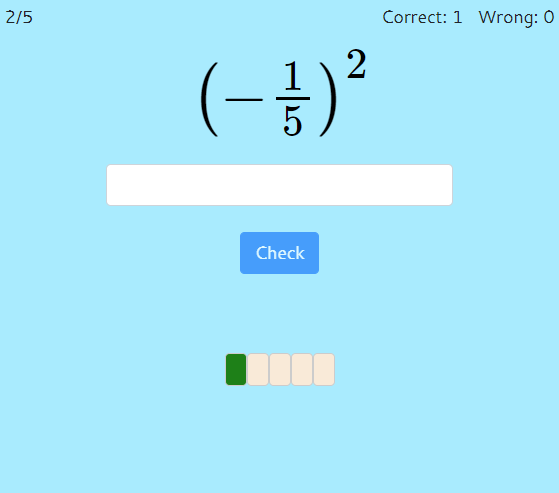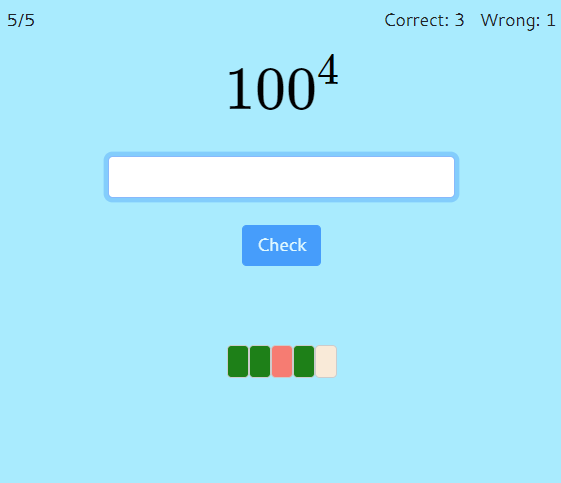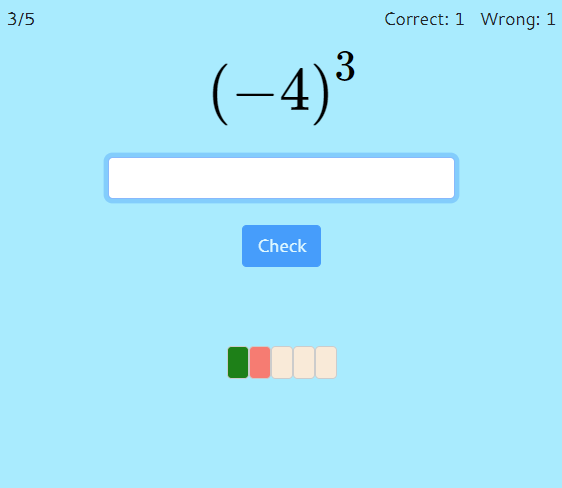MM Practice
×
Multiplication
Division
Place Value
Fractions & Decimals
Measurement
Statistics & Probability
Pre-Algebra
Money
Kindergarten
Geometry

# Exponents and Powers of TenOnline practice for grades 5-8

This page provides online practice for exponents and evaluating powers. For example, you would find the value of $\big(\frac{1}{2}\big)^3$ or $10^6$.

(An exponent is the little elevated number in this expression: $2^4$. It indicates how many times the base number (in our case 2) is multiplied by itself. So, $2^4$ means $2\times2\times2\times2=16$.)

Options for the online practice include positive, zero, and negative exponents; whole-number, fractional, or decimal bases; and positive or negative bases.

You can evaluate powers (expressions with exponents), evaluate expressions where we multiply a power of ten by some factor, or do both.

You can choose timed or untimed practice, and the number of practice problems.

Screenshots from the activity:OR
Practice for a set time: min

Allow my comment to be posted on this site